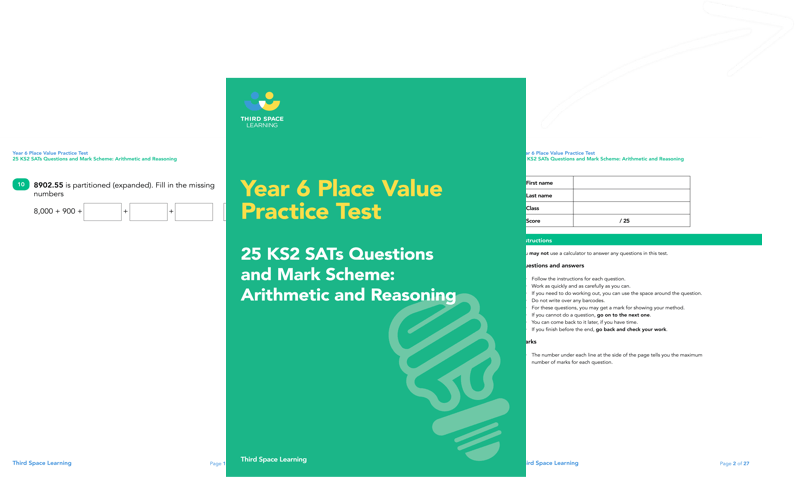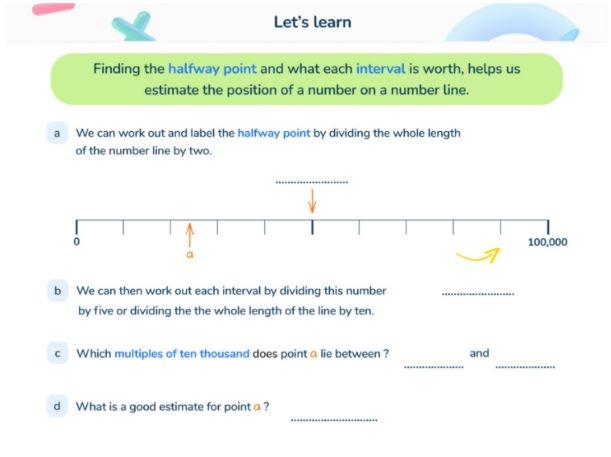Teaching support from the UK’s largest provider of in-school maths tuitionone to one lessonsschools supported

Deepen mathematical understanding

396 mastery-aligned teaching units designed to plug individual gaps and delivered by specialist tutors

See it in action

Hundreds of FREE online maths resources!

Daily activities, ready-to-go lesson slides, SATs revision packs, video CPD and more!Explore all resources
Dictionary & Wiki

Deepen mathematical understanding

396 mastery-aligned teaching units designed to plug individual gaps and delivered by specialist tutors

See it in action

# What Is Estimation in Maths: Estimating Numbers Explained for Primary School Teachers and Parents

Estimation in maths is an important process taught from KS1. It encourages children to have a good understanding of the magnitude of numbers rather than overly relying on mathematical procedures to achieve an answer.

### What is estimating in maths?

Estimating in maths is a way of approximately calculating an answer (getting a ‘rough answer’) to check its accuracy (the ‘right answer’). You shouldn’t need to use a calculator or any written methods when estimating, even with large numbers or decimal numbers.

Estimating numbers will always require you to use skills from rounding numbers in one way or another.

### Examples of estimating calculations

A pencil costs 98p and you want to buy 29 – one for each child in the class. You would estimate that this would cost £29.

A bottle of fizzy drink holds 2 litres and you want to share it amongst 8 friends. You could estimate, by eye, that each friend could get half a glass each (this obviously depends on the capacity of the glass!)KS2 SATs Questions: Place Value

25 Arithmetic and Reasoning maths questions on place value, targeting knowledge of base ten number system and ability to manipulate numbers, especially larger and decimal numbers.Download Free Now!

### When do children learn about estimating in the national curriculum?

Year 2:

• identify, represent and estimate numbers using different representations, including the number line
• choose and use appropriate standard units to estimate and measure length/height in any direction (m/cm); mass (kg/g); temperature (°C); capacity (litres/ml) to the nearest appropriate unit, using rulers, scales, thermometers and measuring vessels

Year 3:

• identify, represent and estimate numbers using different representations
• estimate the answer to a calculation and use inverse operations to check answers
• estimate and read time with increasing accuracy to the nearest minute

Year 4:

• identify, represent and estimate numbers using different representations
• estimate and use inverse operations to check answers to a calculation
• estimate, compare and calculate different measures, including money in pounds and pence

Year 5:

Year 6:

• use estimation to check answers to calculations and determine, in the context of a problem, an appropriate degree of accuracy
• calculate, estimate and compare volume of cubes and cuboidsAn online Third Space Learning maths lesson for Year 6, teaching estimating numbers up to 10 million on an number line.

### How does estimating relate to other areas of maths?

As mentioned above, estimating is referenced throughout the National Curriculum in a variety of topics: using the four operations, measurement and geometry.

### Estimating in real life

We use estimating every day in real life – probably without even noticing. If you are buying a packet of crisps for 99p and a drink for £1.99, you would estimate the total cost to be around £3 (the exact answer would be £2.98).

You might be considering refilling your car with petrol, but if you know how many miles per gallon your car does, and how much petrol you have left, you might be able to estimate how far you can go before refilling.

Estimating is also often used in professional contexts such as in project planning and product development.

### 3 worked examples for estimating in maths

1. Estimate the answer to 3.39 + 5.52.

Estimation questions (especially those without context) often don’t specify the degree of accuracy required in the estimation. The further away we round to, the less accurate we will be.

For example, numbers with 2 decimal places like these could be rounded to 1 decimal place (3.4 + 5.5) to give us an approximate answer of 8.9.

However, they could also be rounded to the nearest whole number (3 + 6) to give us an approximate answer of 9.

Rounding to 1 decimal place gave us a more accurate answer, but was arguably a slightly more complex calculation mentally, whereas rounding to the nearest whole number gave us a less accurate answer, but made simplifying the mental calculation easier.

Note: In KS3 maths and GCSE maths, when estimating and rounding numbers, the amount of ‘significant figures’ they are required to be will often be specified.

2. A phone costs £578.92, but there is a 40% discount. Approximately how much does it cost now?

The word ‘approximately’ is used to mean estimation. As previously mentioned, the amount here could be rounded to £578.90, £579, £580 or £600 – again, the further away it’s rounded, the less accurate the answer will be.

However, looking at the four options we have here and taking the context of the problem into account – money – we could logically conclude that using the last option would be the most straightforward, especially when working with percentages.

40% of £600 is £240, so the phone’s new approximate cost or cost estimate can be calculated using subtraction: £600 – £240 = £360. (The real answer is £347.35, so our approximate answer was a good estimation.)

As you can see, word problems will often require the pupil to consider what the better estimate to use would be.

3. Use rounding to estimate a sensible answer to 120,107 x 61.

120,107 rounds to 120,110 or 120,100 or 120,000 or 100,000.

Of the first four options, the third – 120,000 – would be the best estimate to use as not only is it one of the easiest to multiply by 60 (which is what 61 rounds to) mentally, but it would give a more accurate answer than choosing 100,000. 120,000 x 60 = 7,200,000 (times table knowledge can be used here as 12 x 6 = 72).

### 5 estimating in maths practice questions and answers

1. Layla wants to estimate the answer to this calculation involving fractions.

Answer: 4 – 2 + 2

2. To find the approximate total of 3.2 + 5.9, which two whole numbers would you add together?

3. Megan walks 3,102m on Monday, 4,989m on Tuesday and 2,050m on Wednesday. Approximately how many metres has she walked altogether?

Answer: 3,000m + 5,000m + 2,000m = 10,000m

4. Round 53,892 to the nearest thousand to estimate the answer to 53,892 ÷ 6.

Answer: 54,000 ÷ 6 = 9,000

5. Estimate 532 × 12 to help you to identify which of the following is the actual answer to the calculation: 638; 6,384; 63,840; 1,596

Answer: 530 x 10 = 5,300 which is closest to 6,384

### FAQs

How do you do estimation in maths?

Estimating in maths requires a strong knowledge of rounding as that is often the best way to ensure you don’t make a mistake in your estimation. For example, if asked to estimate the answer for 0.7 x 0.8, by rounding the decimals and then multiplying (0.7 and 0.8 both round up to 1, and 1 x 1 = 1) you are better able to check the accuracy of the answer (5.6 is much larger than 1, so the multiplication could be recalculated to achieve 0.56, which is much closer to 1).

What are the steps to estimate answers?

Firstly, establish how accurate the estimate needs to be (to the nearest penny? Tenths? Hour? Gram? Kilometre?). Next in the estimation process would be to round the numbers up or down, depending on the context of the question.

How do you explain estimation to children?

Estimating is a way of getting a ‘rough answer’ by using rounding. You shouldn’t need to use a calculator or any written methods when estimating.

Wondering about how to explain other key maths vocabulary to your children? Check out our Primary Maths Dictionary and our What is Maths Mastery? blog to help with lesson planning and at home maths revision.

You can find plenty of printable estimating worksheets, numeracy worksheets and plenty of other learning resources for primary school pupils on the Third Space Learning Maths Hub.

Do you have pupils who need extra support in maths?
Every week Third Space Learning’s maths specialist tutors support thousands of pupils across hundreds of schools with weekly online 1-to-1 lessons and maths interventions designed to plug gaps and boost progress.

Since 2013 we’ve helped over 150,000 primary and secondary school pupils become more confident, able mathematicians. Learn more or request a personalised quote for your school to speak to us about your school’s needs and how we can help.

Primary school tuition targeted to the needs of each child and closely following the National Curriculum.##### Sophie Bartlett
Year 6 Teacher
Sophie teaches mixed age classes at a small school in central England. She is a self confessed grammar pedant and number nerd so we've welcomed her as a regular author and editor for Third Space Learning.

Related Articles

x#### KS2 SATs Questions: Place Value

25 Arithmetic and Reasoning maths questions on place value, targeting knowledge of base ten number system and ability to manipulate numbers, especially larger and decimal numbers.

#### KS2 SATs Questions: Place Value25 Arithmetic and Reasoning maths questions on place value, targeting knowledge of base ten number system and ability to manipulate numbers, especially larger and decimal numbers.